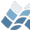NV5 GEOSPATIAL SOFTWARECHEBCOEF

CHEBCOEF

## Author

Craig B. Markwardt, NASA/GSFC Code 662, Greenbelt, MD 20770
craigm@lheamail.gsfc.nasa.gov
UPDATED VERSIONs can be found on my WEB PAGE:
http://cow.physics.wisc.edu/~craigm/idl/idl.html

## Purpose

Estimate Chebyshev polynomial coefficients of a function on an interval

## Major Topics

Curve and Surface Fitting

## Calling Sequence

p = CHEBCOEF(FUNC, PRIVATE, FUNCTARGS=functargs, /DOUBLE, /EXPRESSION, \$
PRECISION=prec, ERROR=err, NMAX=nmax, INTERVAL=interval, \$
REDUCE_ALGORITHM=, STATUS=)

## Description

CHEBCOEF estimates the coefficients for a finite sum of Chebyshev
polynomials approximating the function FUNC(x) over an interval.
The user can choose the desired precision and maximum number of
chebyshev coefficients.
This routine is intended for functions which can be evaluated to
full machine precision at arbitrary abcissae, and which are smooth
enough to ensure that the coefficients are a decreasing sequence.
For already-tabulated or potentially noisy data, the routines
CHEBGRID or CHEBFIT should be used instead.
The function to be approximated may either be the name of an IDL
function (the default behavior), or an IDL expression (using the
/EXPRESSION keyword).
The procedure uses a modified form of the classic algorithm for
determining the coefficients, which relies the orthogonality
relation for Chebyshev polynomials. The interval [a,b] is
subdivided successively into sets of subintervals of length
2^(-k)*(b-a),(k = 0,1,2...). After each subdivision the
orthogonality properties of the Chebyshev polynomials with respect
to summation over equally-spaced points are used to compute two
sets of approximate values of the coefficients cj, one set
computed using the end-points of the subintervals, and one set
using the mid-points. Certain convergence requirements must be
met before terminating. If the routine fails to converge with 64
coefficents, then the current best-fitting coefficients are
returned, along with an error estimate in the ERROR keyword.
CHEBCOEF never returns more than 64 coefficients.
The coefficients may be further refined. If the keyword
REDUCE_ALGORITHM is set to a value of 1, then any high order
coefficients below a certain threshold are discarded. If
REDUCE_ALGORITHM is set to 2 (the default), then all coefficients
below the threshold are discarded rather than just the high order
ones. The threshold is determined by the PRECISION keyword.

## Inputs

FUNC - a scalar string, the name of the function to be
approximated, or an IDL string containing an expression to
be approximated (if /EXPRESSION is set).
PRIVATE - any optional variable to be passed on to the function to
be integrated. For functions, PRIVATE is passed as the
second positional parameter; for expressions, PRIVATE can
be referenced by the variable 'P'. CHEBCOEF does not
examine or alter PRIVATE.

## Returns

An array of Chebyshev coefficients which can be passed to
CHEBEVAL. NOTE: the convention employed here is such that the
constant term in the expansion is P(0)*T0(x) (i.e., the convention
of Luke), and not P(0)/2 * T0(x).

## Keyword Parameters

DOUBLE - if set, then computations are done in double precision
rather than single precision.
ERROR - upon return, this keyword contains an estimate of the
maximum absolute error in the approximation.
EXPRESSION - if set, then FUNC is an IDL expression to be
approximated, rather than the name of a function.
FUNCTARGS - A structure which contains the parameters to be passed
to the user-supplied function specified by FUNCT via
the _EXTRA mechanism. This is the way you can pass
using common blocks. By default, no extra parameters
are passed to the user-supplied function.
INTERVAL - a 2-element vector describing the interval over which
the polynomial is to be evaluated.
Default: [-1, 1]
NMAX - a scalar, the maximum number of coefficients to be
estimated. This number may not exceed 64.
Default: 64
PRECISION - a scalar, the requested precision in the
approximation. Any terms which do not contribute
significantly, as defined by this threshold, are
discarded. If the function to be estimated is not
well-behaved, then the precision is not guaranteed to
reach the desired level. Default: 1E-7
REDUCE_ALGORITHM - a scalar integer, describes how insignificant
terms are removed from the fit. If 0, then all terms
are kept, and none are dicarded. If 1, then only
trailing terms less than PRECISION are discarded. If
2, then both trailing and intermediate terms less than
Default: 2
STATUS - upon return, this keyword contains information about the
status of the approximation. A value of -1 indicates bad
input values; a value of 0 indicates the required
accuracy was not obtained; a value of 1 indicates
success.

## Example

x = dindgen(1000)/100 ; Range of 0 to 10
p = chebcoef('COS(x)', /expr, interval=[0d, 10d]) ;; Compute coefs
y = chebeval(x, p, interval=[0d,10d]) ;; Eval Cheby poly
plot, x, y - cos(x) ; Plot residuals

## References

Abramowitz, M. & Stegun, I., 1965, *Handbook of Mathematical
Functions*, 1965, U.S. Government Printing Office, Washington,
D.C. (Applied Mathematical Series 55)
CERN, 1995, CERN Program Library, Function E406
Luke, Y. L., *The Special Functions and Their Approximations*,